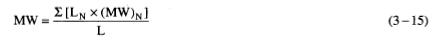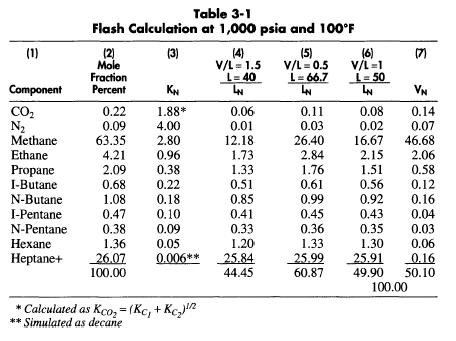# Liquid Molecular Weight

The molecular weight of the liquid stream is calculated from the weighted average liquid component molecular weight given by:This is calculated in Table 3-3. Column 2 is as in Table 3-2 and Column 3 is the liquid stream composition for 100 moles of feed as calculated in Table 3-1, Column 7. Column 4 is Column 2 times Column 3 and represents the weight of each component in the liquid stream. The molecular
weight of the liquid is: